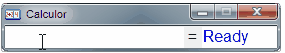﻿ Variables

# Variables

Navigation:  »No topics above this level«

# VariablesEnter topic text here.

Variables

You can use variables in your expressions.

Here is how to define a variable:

a:=5;

You use := to assign a variable, and you end the assignment with a ;

Then you just type your expression as you like, and a will be calculated throughout the expression.

Now, anywhere you use a in the expression, it will be interpreted as it's value.

You can even assign multiple variables.

then use them like this:

a:=5; 2+a                        = 7

a:=2; 2*a-40+a                = -34

a:=3;b:=2; 2*b+(a*a)        = 13

a:=5; 2*a*(2*2)2                  = 80